Related Articles

# How to Rotate X-Axis Tick Label Text in Matplotlib?

• Last Updated : 24 Jan, 2021

Matplotlib is an amazing and one of the most widely used data visualization library in Python for plots of arrays. It is a multi-platform data visualization library built on NumPy arrays and designed to work with the broader SciPy stack. It is much popular because of its customization options as we can tweak about any element from its hierarchy of objects.

### Rotating X-axis labels

To rotate X-axis labels, there are various methods provided by matplotlib i.e. change it on the Figure-level or by changing it on an Axes-level or individually by using built-in functions. Some methods are listed below :

Let’s create a simple line plot which we will modify in the further examples:

## Python3

 `# Import libraries``import` `matplotlib.pyplot as plt``import` `numpy as np`` ` `# Creating dataset``x ``=` `np.arange(``0``, np.pi``*``2``, ``0.05``)``y ``=` `np.sin(x``*``*``2``)`` ` `# Creating plot``plt.plot(x, y)`` ` `# Setting title``plt.title(``'Simple Example'``)`` ` `# Show plot``plt.show()`

Output :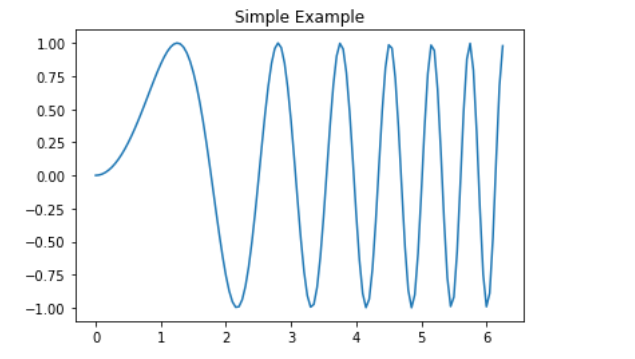Example 1: In this example, we will rotate X-axis labels on Figure-level using plt.xticks().

Syntax: matplotlib.pyplot.xticks(ticks=None, labels=None, **kwargs)

Parameters: This method accept the following parameters that are described below:

• ticks: This parameter is the list of xtick locations. and an optional parameter. If an empty list is passed as an argument then it will removes all xticks
• labels: This parameter contains labels to place at the given ticks locations. And it is an optional parameter.
• **kwargs: This parameter is Text properties that is used to control the appearance of the labels.

## Python3

 `# Import libraries``import` `matplotlib.pyplot as plt``import` `numpy as np`` ` `# Creating dataset``x ``=` `np.arange(``0``, np.pi``*``2``, ``0.05``)``y ``=` `np.sin(x``*``*``2``)`` ` `# Creating plot``plt.plot(x, y)`` ` `# Rotating X-axis labels``plt.xticks(rotation ``=` `25``)`` ` `# Setting title``plt.title(``'Rotating using plt.xticks()'``)`` ` `# Show plot``plt.show()`

Output :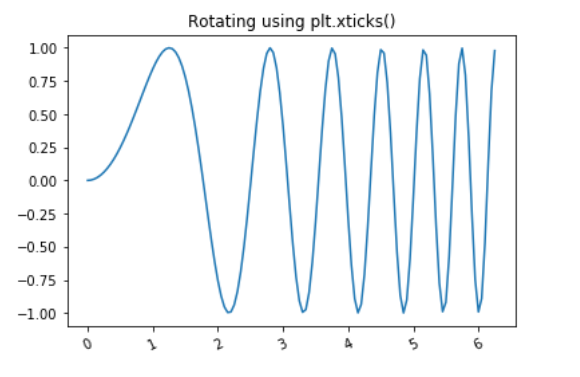Example 2: In this example, we will rotate X-axis labels on Axes-level using tick.set_rotation().

Syntax: Axes.get_xticks(self, minor=False)

Parameters: This method accepts the following parameters.

• minor : This parameter is used whether set major ticks or to set minor ticks

Return value: This method returns a list of Text values.

## Python3

 `# Import libraries``import` `matplotlib.pyplot as plt``import` `numpy as np`` ` `# Creating dataset``x ``=` `np.arange(``0``, np.pi``*``2``, ``0.05``)``y ``=` `np.sin(x``*``*``2``)`` ` `# Creating Figure``fig, ax ``=` `plt.subplots() `` ` `# Creating plot``ax.plot(x, y)`` ` `# Rotating X-axis labels``ax.set_xticklabels(ax.get_xticks(), rotation ``=` `50``)`` ` `# Setting title``plt.title(``'Rotating using tick.set_rotation()'``)`` ` `# Show plot``plt.show()`

Output :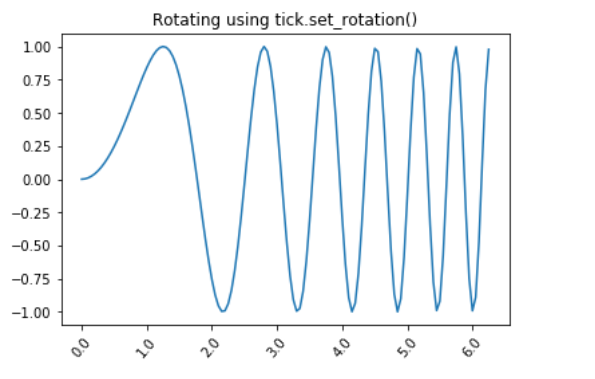Example 3: In this example, we will rotate X-axis labels individually by using built-in ax.set_xticklabels() function.

## Python3

 `# Import libraries``import` `matplotlib.pyplot as plt``import` `numpy as np`` ` `# Creating dataset``x ``=` `np.arange(``0``, np.pi``*``2``, ``0.05``)``y ``=` `np.sin(x``*``*``2``)`` ` `# Creating Figure``fig, ax ``=` `plt.subplots() `` ` `# Creating plot``ax.plot(x, y)`` ` `# Rotating X-axis labels``for` `tick ``in` `ax.get_xticklabels():``    ``tick.set_rotation(``75``)`` ` `# Setting title``plt.title(``'Rotating using ax.set_xticklabels()'``)`` ` `# Show plot``plt.show()`

Output :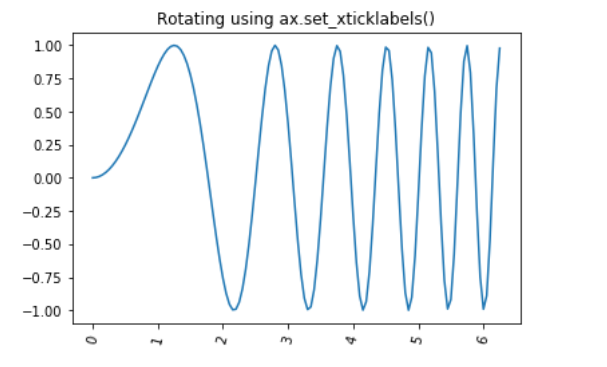Example 4: In this example, we will rotate X-axis labels individually by using built-in ax.xtick_params() function.

Syntax: matplotlib.pyplot.tick_params(axis=’both’, **kwargs)

## Python3

 `# Import libraries``import` `matplotlib.pyplot as plt``import` `numpy as np`` ` `# Creating dataset``x ``=` `np.arange(``0``, np.pi``*``2``, ``0.05``)``y ``=` `np.sin(x``*``*``2``)`` ` `# Creating Figure``fig, ax ``=` `plt.subplots() `` ` `# Creating plot``ax.plot(x, y)`` ` `# Rotating X-axis labels``ax.tick_params(axis``=``'x'``, labelrotation ``=` `100``)`` ` `# Setting title``plt.title(``'Rotating using ax.xtick_params()'``)`` ` `# Show plot``plt.show()`

Output :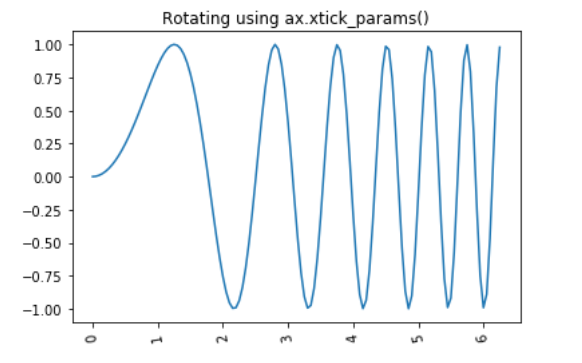Attention geek! Strengthen your foundations with the Python Programming Foundation Course and learn the basics.

To begin with, your interview preparations Enhance your Data Structures concepts with the Python DS Course. And to begin with your Machine Learning Journey, join the Machine Learning – Basic Level Course

My Personal Notes arrow_drop_up